Home.

This is a detailed explanation of how the pips were designed, and how their sizes for the number cards were derived. Assumed is that all dimensions are in millimeters, and cards are 62.5 wide and 88.39 high.

Each pip starts with a basic regular polygon, highlighted in red in figure X1 below. Point G is one corner of the basic polygon. Point A is the midpoint of one side, while point F is the center. Points B, C, D, and E are evenly spaced between A and F. Within any one pip, triangles GAB, GBC, GCD, GDE, and GEF all have the same area.

The H-F distance is 120% of the G-F distance, and the I-F distance is 140% of the G-F distance.

 figure X1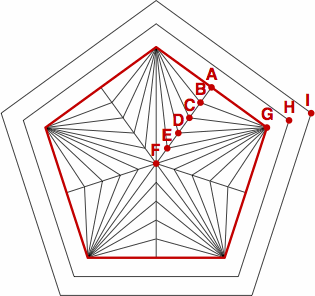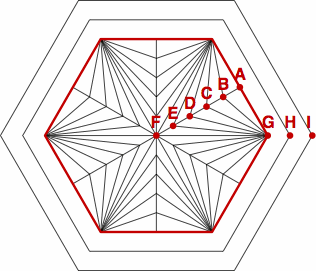Then to produce the pips, selected areas are filled in:

 figure X2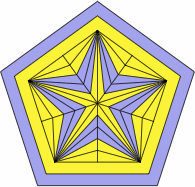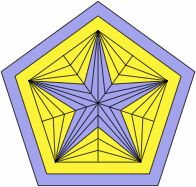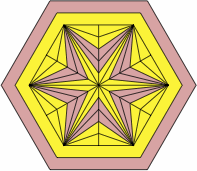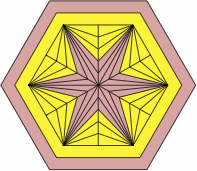The pip sizes for the number cards are in the table below. This diagram shows exactly what is being measured. Figure X3 is an excerpt from that diagram, for the 5-point pip that appears on a number card of rank 8:

figure X3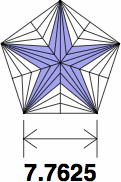s = number of sides 3 4 5 6 suggested pip sizes for the number cards 12.8137 8.4319 6.4284 5.2312 4.4232 3.8373 13.0817 8.6083 6.5628 5.3406 4.5157 3.9175< 13.3758 8.8018 6.7104 5.4607 4.6172 4.0056 13.7009 9.0157 6.8734 5.5934 4.7295 4.1030 14.0632 9.2541 7.0552 5.7413 4.8545 4.2115 14.4712 9.5226 7.2599 5.9078 4.9954 4.3336 14.9359 9.8284 7.4930 6.0975 5.1558 4.4728 15.4731 10.1818 7.7625 6.3169 5.3412 4.6337 16.1055 10.5980 8.0798 6.5750 5.5595 4.8230 16.8678 11.0996 8.4622 6.8862 5.8227 5.0513 17.8161 11.7236 8.9380 7.2734 6.1500 5.3353 19.0496 12.5353 9.5568 7.7770 6.5758 5.7047 20.7667 13.6652 10.4182 8.4780 7.1685 6.2189 23.4528 15.4328 11.7658 9.5746 8.0958 7.0233 28.8738 19.0000 14.4854 11.7877 9.9670 8.6467 38.0 × exp (−0.3 × log (p)) × sqrt (tan (180° ÷ s) ÷ s) The numbers 38.0 and −0.3 werearbitrarily chosen to give attractive results.

Pip sizes for the ace cards are 15% smaller in linear measurement than their number-card equivalents, with A corresponding to 1, B to 2, et cetera. The smaller size allows room for the border. The number 15% was chosen arbitrarily.